<img alt="" src="https://secure.perk0mean.com/171547.png" style="display:none;">

# Multiple Forest Plots and the use of SAS MacrosThis blog post discusses the SAS/Graph Annotation option and how this can be used in combination with SAS Macros to allow the creation of multiple Forest Plots, giving details on what can and cannot be controlled as part of the macro call. The purpose of this paper is to highlight the methods of using the ANNOTATE Option available with the SAS/GRAPH procedures for producing a Forest Plot using the SAS system and how this can be adapted to allow multiple plots to be created using SAS Macros.

SAS Programmers are frequently asked to produce graphical representations of data, from simple pie charts and box plots to more statistical graphics such as the Forest Plot. SAS/Graph provides graphing tools to create plots and charts with only a few SAS statements and by using the built in options provided it is possible to produce graphical summaries of almost any need. However the default graphs do not always suffice and it is occasionally necessary to ‘add’ to the default outputs.

In these cases, SAS/Graph’s Annotate data set can be used to overcome the limitations of SAS/Graph’s procedures to create a new type of graph from an existing one or to enhance the normal output of a graph. Forest Plots are an example of this in that there is no single method of creating such a plot using the SAS system and the use of the Annotate dataset is necessary.

The Annotate data set can be used to add additional information to an existing graphical output or to create a completely new type of graph. The Annotate dataset allows the user to place lines, bars, text or symbols on a graph with the positioning of these additions given using either the data from the graphed data set itself as a reference, or with coordinates provided by the programmer. Usually, the annotation uses a combination of the two.

THE FOREST PLOT

A Forest Plot is a graphical display designed to illustrate the strength of treatment effects across treatments groups, subgroups of a study and multiple studies addressing the same question. The Plots were initially developed as a means of graphically representing a meta-analysis of the results of randomized controlled trials.

Although Forest Plots can take several forms, they are commonly presented with two columns. The left-hand column lists the names of the analysis groups and the right-hand column is a plot of the measure of effect (e.g. an odds ratio) for each of these groups (often represented by a circle) incorporating confidence intervals represented by horizontal lines.

The advantage of using a forest plot to show such data is that the reviewer can see at a glance any differences between several analysis groups without having to look at the results in detail – saving time.

An example of a Forest Plot is given below (Figure 1) – Subgroup analysis names have been removed but you can see that the confidence Intervals and the hazard ratios are clearly shown and allow a quick comparison of the subgroups. In this example some Hazard Ratios and Confidence Intervals are not shown due to the number of events for that subgroup being to low to calculate an accurate Hazard ratio.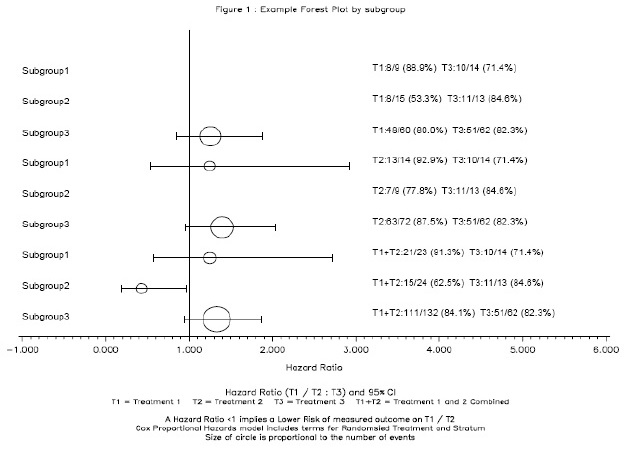Figure 1 has been created using the bubble option of PROC GPLOT and as a result the circles representing the Hazard Ratios are part of the default output. However the Subgroup labels, the Confidence Intervals and the Text showing the number of events within each subgroup have all been added to the default output using the Annotate option and an Annotate Dataset.

ANNOTATE DATASET

The Annotate option requires a dataset specifically designed to tell SAS/GRAPH how to enhance your current output. There are two basic functions which allow for moving and drawing, and other functions can be used to position labels, create bars, pies and polygons. The ANNOTATE dataset itself must have one observation per function and essentially tells SAS what and where to draw or place text.

The FUNCTION variable created in the dataset determines the graphics element that is drawn. The elements available are given in Table 1. Any of the elements given in Table 1 can be used to create a Figure. For the Forest Plot shown in Figure 1 we need only use the Move, Draw and Label elements to create the Confidence intervals and the text that is shown on the graph. The Hazard ratio bubbles are created as default and so no annotation is required for this.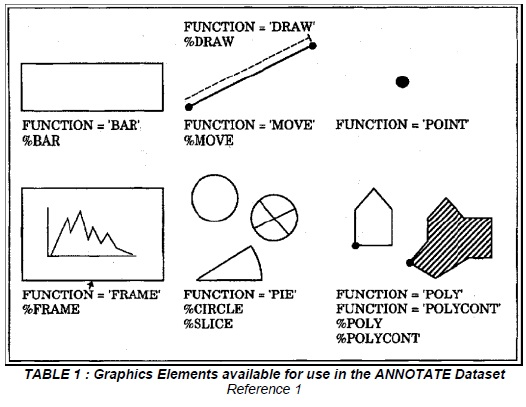A simple example (showing the creation of the Confidence Intervals for Figure 1 above) is shown below. You can see that the code uses the data set that is to be graphed as a basis and adds the functions move and draw to tell SAS where to put the Confidence Intervals. The Confidence Intervals and hazard ratios have already been calculated and are stored in the dataset data1. The bars at the end of the Confidence Intervals are created separately to the actual Confidence Intervals.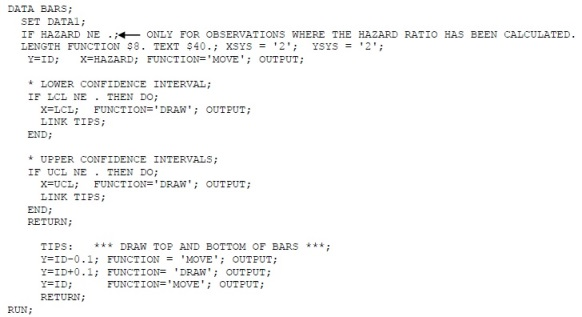The text on the graph is created using the label element function an example of which is given below.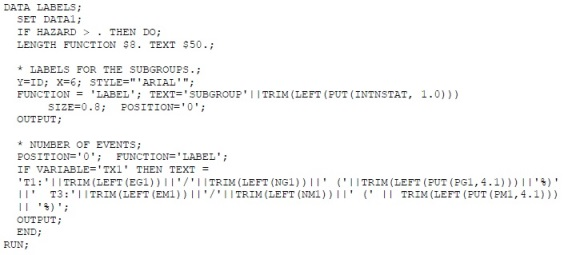Each subgroup requires its own label function and so the code above would have several more label statements for the number of events.

The Confidence Interval and Label annotation datasets can then be set together to create a single annotation dataset which is then referenced within the graphical procedure as ANNOTATE= dataset name.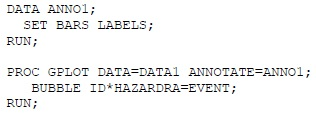Axis, titles and footnote statements can be added to the GPLOT procedure as normal.

MACROS

Due to the amount of detail required in the Annotate dataset programmers tend to prefer to modify the code for each output required. However sometimes ten’s maybe hundred’s of figures are required and so a macro would be the most efficient method to use.

The changes take place throughout the code including the code which creates the annotate dataset and dependant upon the changes across figures are quite minimal. All the cosmetics of the figure are controllable and the annotate dataset is created from your actual data and so can be adapted within a macro taking away the need for separate codes.

SOURCES

Reference 1 – www.sas.com## Related Articles#### How to Deal with Large SAS Datasets in Clinical Trials

by Clinical Programming Team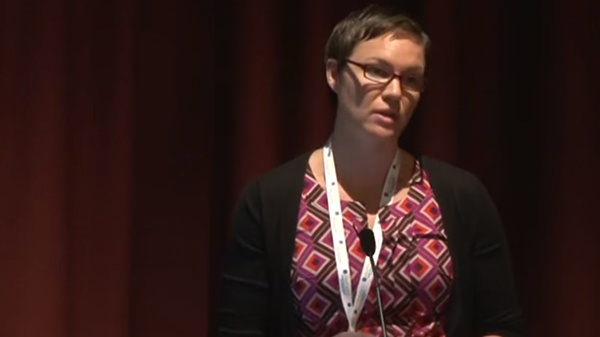#### Efficient Data Reviews and Quality in Clinical Trials [Video]

by Statistical Consultancy Team